HOME COURSES PREVIEW REVIEW ABOUT CONTACTToll-Free Info & Ordering M-F: 9am-5pm (PST): (877) RAPID-1024/7 Technical SupportQUICK TOURMember Login:Rapid Courses Catalog Mathematics in 24 Hours Chemistry in 24 Hours Biology in 24 Hours Physics in 24 HoursMath Survival Weekly
Get the insider's tips and tricks in how to survive your math course and ace the next test. Subscribe the Web's only math weekly newsletter for students and learn:
- How to Study Math Effectively
- How to Take Math Courses Strategically
- How to Solve Math Problems Systematically
- How to Score High in Math Exams
- How to Master Math Rapidly

Enter your name and email below and get started today!Math Study Lounge These study sheets are for quick review on the subjects. Refer to our rapid courses for comprehensive review.     - Getting Started with Algebra     - Geometry Basics     - How to Solve Math Problems     - Trigonometry Quick Review     - Statistics At-A-Glance     - Calculus PreviewHome » Calculus

Functions and Graphs

 Topic Review on "Title": Function (from a set X to a set Y): A correspondence that associates with each element x (independent variable) of X and a unique element y (dependent variable) of Y. Notation: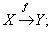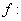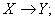or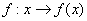. Domain of a function: The data set of all real numbers for which the correspondence makes sense. One to one functions: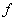is a function from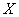to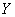. Increasing functions: If S is a subset of X and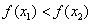whenever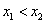, in S, thenis an increasing function in S. Decreasing functions: If S is subset of X and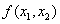whenever, in S, thenis a decreasing function in S. Slope: If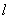is a line which is not parallel to the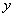-axis and if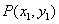and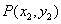are distinct points on, then the slope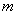ofis given by: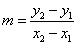. Equation of a curve: Suppose of a curve composed of points whose coordinates are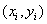for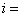1, 2,…. If there is an equation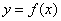, by which all the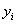can be calculated through substituting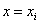, the equationis called the equation of the curve. Equation of a line: If the slope of a line is given (denoted by) and if a point on the line is given (coordinate is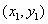) , the line equation would be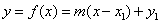. Vertical shifts: If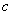is a real number, the graph of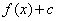is the graph ofshifted upwardunits for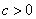or shifted downward for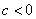. Horizontal shifts: Ifis a real number, the graph of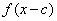is the graph ofshifted to the rightunits foror shifted to the leftunits for. Reflection in the y-axis: The graph of the function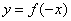is the graph ofreflected in the y-axis. Reflection in the x-axis: The graph of the function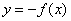is the graph ofreflected in the x-axis. Vertical stretching and shrinking: Ifis a real number, the graph of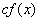is the graph ofstretched vertically byfor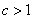or shrunk vertically byfor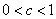. Horizontal stretching and shrinking: Ifis a real number, the graph of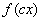is the graph ofstretched horizontally byforor shrunk horizontally byfor. Composite functions: Ifis a function fromtoand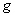is the function fromto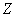, then the composite function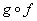is the function fromtodefined by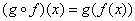. Inverse functions: Letbe a one to one function fromto. Then, a functionfromtois called the inverse function ofif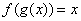for all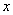inand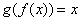for allin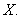Rapid Study Kit for "Title":
 Flash Movie Flash Game Flash Card Core Concept Tutorial Problem Solving Drill Review Cheat Sheet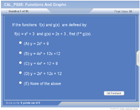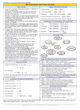"Title" Tutorial Summary : This tutorial covers functions and their characteristics. The concept of graphing a function allows for a discussion of the inverse, composite and transformation of functions to be handled at a more manageable level. A function is defined in its traditional way with the help of set notation and one to one correspondence. The domain of a function is needed to be discussed so the inverse of a function can be covered. Transformations of functions are introduced with the assistance of graphs and special notation.

 Tutorial Features: Specific Tutorial Features: Graph of functions, ordered pair of functions, and functions are introduced with step by step examples. Sketches of functions with visual aids are shown in the tutorial to help introduce concepts such as decreasing functions. Series Features: Concept map showing inter-connections of new concepts in this tutorial and those previously introduced. Definition slides introduce terms as they are needed. Visual representation of concepts Animated examples—worked out step by step A concise summary is given at the conclusion of the tutorial.

 "Title" Topic List: `Functions Two sets and their correspondence Functions and their definition Domain of functions One to one functions Ordered pair of a functionIncreasing and decreasing functionsTwo dimensional linear functions Definition of slope Equation of a lineParent Functions Definition of parent functionsTransformations of functions Vertical shifts Horizontal shifts Reflection in the y-axis Reflection in the x-axis Vertical stretching and shrinking Horizontal stretching and shrinkingComposite and inverse functions`

See all 24 lessons in Calculus, including concept tutorials, problem drills and cheat sheets:
Teach Yourself Calculus Visually in 24 Hours

 ©2014 Rapid Learning Center. Privacy Policy | Disclaimer Chemistry Survival Publishing, Biology Survival Publishing and Physics Survival Publishing are the divisions of Rapid Learning Inc.